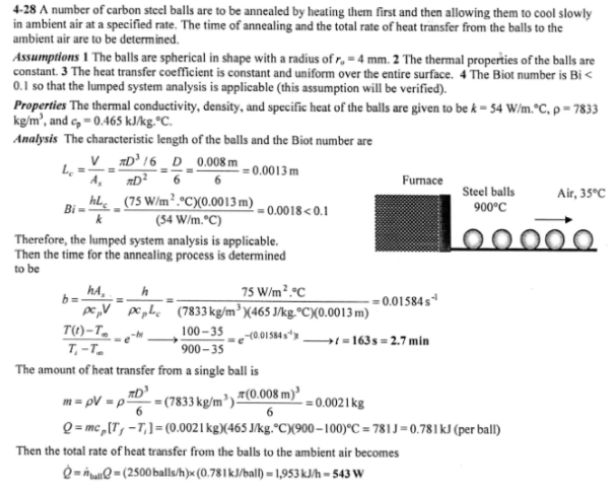heat and mass transfer problems engineering equations heat and mass transfer school homework engineering heat and mass transfer formulas heat and mass transfer solutions to heat and mass transfer problems full solution engineering problem solution heat and mass transfer math problems engineering equations heat and mass transfer school homework engineering solutions to heat and mass transfer formulas heat problems mass problem solutions to transfer problems full solution heat and mass transfer
heat and mass transfer problems engineering equations heat and mass transfer school homework engineering heat and mass transfer formulas heat and mass transfer solutions to heat and mass transfer problems full solution engineering problem solution heat and mass transfer math problems engineering equations heat and mass transfer school homework engineering solutions to heat and mass transfer formulas heat problems mass problem solutions to transfer problems full solution heat and mass transfer
Highalphabet Home Page heat and mass transfer problem solutions Heat and Mass Transfer Page
Carbon steel balls (rho=7833 kg/m^3, k=54 W/mK, c_p=0.465 kJ/kg C, and alpha=1.474x10^-6 m^2/s) 8 mm in diameter are annealed by heating them first to 900 C in a furnace and then allowing them to cool slowly to 100 C in ambient air at 35 C. If the average heat transfer coefficient is 75 W/m^2 K, determine how long the annealing process will take. If 2500 balls are to be annealed per hour, determine the total rate of heat transfer from the balls to the ambient air.Carbon steel balls (rho=7833 kg/m^3, k=54 W/mK, c_p=0.465 kJ/kg C, and alpha=1.474x10^-6 m^2/s) 8 mm in diameter are annealed by heating them first to 900 C in a furnace and then allowing them to cool slowly to 100 C in ambient air at 35 C. If the average heat transfer coefficient is 75 W/m^2 K, determine how long the annealing process will take. If 2500 balls are to be annealed per hour, determine the total rate of heat transfer from the balls to the ambient air.